Using the ‘DiffXTables’ R package to detect heterogeneity

Created October 22, 2019; Updated May 14, 2021; November 10, 2019; March 19, 2020

The heterogeneity question asks whether a relationship between two random variables has changed across conditions. It is often fundamental to a scientific inquiry. For example, a biologist could ask whether the relationship between two genes has been modified in a cancer cell from that in a normal cell. The ‘DiffXTables’ R package answers the heterogeneity question via evaluating statistical evidence for distributional changes in the involved variables from the observed data.

Given multiple contingency tables of the same dimension, ‘DiffXTables’ offers three methods cp.chisq.test(), sharma.song.test(), and heterogenity.test() to test whether the distributions underlying the tables are different. All three tests use the chi-squared distribution to calculate a p-value to indicate the statistical significance of any detected difference across the tables. However, these tests behave sharply different for various types of pattern heterogeneity present in the input tables. Here, we define pattern types, explain the three tests, and illustrate their similarities and differences by examples. These examples reveal inadequacy of the current textbook solution to the contingency table heterogeneity question.

Types of pattern

A pattern is a contingency table tabulating the counts or frequencies observed for a pair of discrete random variables. We study the distributional differences across tables collected from more than one conditions.

• First-order differential patterns: Some or all pairs of input tables differ in either row or column marginal distributions. Other pairs share the same underlying joint distribution.

• Second-order differential patterns: Some or all pairs of input tables differ in joint distributions not attributed to any difference in marginal distributions.

• Differential patterns: Some or all pairs of tables differ in joint distributions.

• Conserved patterns: All tables share the same joint distributions.

The three tests of distributional differences across tables

The input to all three tests is two or more contingency tables. The output is chi-squared test statistics, their degrees of freedom, and p-values. They also share the same null hypothesis H0 that all tables are conserved in distributions. However, these tests answer distinct alternative hypotheses.

1. The comparative chi-squared test

Alternative hypothesis H1: Patterns represented by the tables are differential.

The statistical foundation of this test is first established in <doi:10.1093/nar/gku086> and the test is then extended to identify differential patterns in networks <doi:10.1093/nar/gkv358>.

It is implemented as the R function cp.chisq.test() in this package.

2. The Sharma-Song test

Alternative hypothesis H2: Patterns represented by the tables are second-order differential.

The test detects differential departure from independence via second-order difference in the joint distributions underlying two or more contingency tables. The test is fully described in (Sharma et al. 2021) <doi:10.1093/bioinformatics/btab240>.

It is implemented as the R function sharma.song.test() in this package.

3. The heterogeneity test

Alternative hypothesis H1: Patterns represented by the tables are differential.

This test is described in (Zar, 2010). Although it widely appears in textbooks, we demonstrate that it is not always powerful in some examples below.

It is implemented as the R function heterogenity.test() in this package.

Examples to illustrate differences among the three tests

Here, we show some examples to demonstrate the usage, similarity and difference between the three tests. All these examples represent strong patterns so that the presence of a pattern type is evident. Both the comparative chi-squared test and the Sharma-Song test perform correctly on all five examples; while the heterogeneity test fails on two examples.

require(FunChisq)
require(DiffXTables)

Example 1: Input tables are conserved. At $$\alpha=0.05$$, all tests perform correctly by not rejecting the null hypothesis of conserved patterns.

tables <- list(
matrix(c(
14,  0,  4,
0,  8,  0,
4,  0, 12), byrow=TRUE, nrow=3),
matrix(c(
7,  0,  2,
0,  4,  0,
2,  0,  6), byrow=TRUE, nrow=3)
)
par(mfrow=c(1,2), cex=0.5, oma=c(0,0,2,0))
plot_table(tables[], highlight="none", xlab=NA, ylab=NA)
plot_table(tables[], highlight="none", xlab=NA, ylab=NA)
mtext("Conserved patterns", outer = TRUE)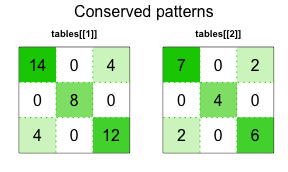cp.chisq.test(tables)
#>
#>  Comparative Chi-Squared Test for Pattern Difference
#>
#> data:  tables
#> X-squared = -1.4211e-14, df = 4, p-value = 1

sharma.song.test(tables)
#>
#>  Sharma-Song Test for Second-Order Differential Contingency Tables Null
#>  table marginal is observed
#>
#> data:  tables
#> X-squared = 2.9934e-32, df = 4, p-value = 1

heterogeneity.test(tables)
#>
#>  Heterogeneity Test for Pattern Difference
#>
#> data:  tables
#> X-squared = 0, df = 4, p-value = 1

Example 2: Input tables are only first-order differential. At $$\alpha=0.05$$, cp.chisq.test() performs correctly by declaring differential patterns; sharma.song.test() performs correctly by not declaring second-order differential patterns; and heterogenity.test() performs incorrectly by not declaring the tables as differential.

tables <- list(
matrix(c(
16, 4, 20,
4, 1,  5,
20, 5, 25), nrow = 3, byrow = TRUE),
matrix(c(
1, 1,  8,
1, 1,  8,
8, 8, 64), nrow = 3, byrow = TRUE)
)
par(mfrow=c(1,2), cex=0.5, oma=c(0,0,2,0))
plot_table(tables[], highlight="none", col="cornflowerblue", xlab=NA, ylab=NA)
plot_table(tables[], highlight="none", col="cornflowerblue", xlab=NA, ylab=NA)
mtext("First-order differential patterns", outer = TRUE)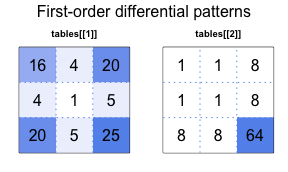cp.chisq.test(tables)
#>
#>  Comparative Chi-Squared Test for Pattern Difference
#>
#> data:  tables
#> X-squared = 49.846, df = 4, p-value = 3.888e-10

sharma.song.test(tables)
#>
#>  Sharma-Song Test for Second-Order Differential Contingency Tables Null
#>  table marginal is observed
#>
#> data:  tables
#> X-squared = 0, df = 4, p-value = 1

heterogeneity.test(tables)
#>
#>  Heterogeneity Test for Pattern Difference
#>
#> data:  tables
#> X-squared = 3.1058, df = 4, p-value = 0.5403

Example 3: Input tables are only first-order differential. At $$\alpha=0.05$$, cp.chisq.test() correctly declares differential patterns; sharma.song.test() performs correctly by not declaring second-order differential patterns; and heterogenity.test() correctly declares differential patterns.

tables <- list(
matrix(c(
8,  1, 1, 38, 4,
5,  1, 1, 17, 1,
2,  1, 1,  9, 1,
2,  1, 1,  4, 1), nrow=4, byrow = TRUE),
matrix(c(
1,  2, 1,  1, 2,
2,  9, 1,  1, 4,
2, 13, 1,  1, 1,
3, 45, 2,  1, 7), nrow=4, byrow = TRUE)
)
par(mfrow=c(1,2), cex=0.5, oma=c(0,0,2,0))
plot_table(tables[], highlight="none", col="cornflowerblue", xlab=NA, ylab=NA)
plot_table(tables[], highlight="none", col="cornflowerblue", xlab=NA, ylab=NA)
mtext("First-order differential patterns", outer = TRUE)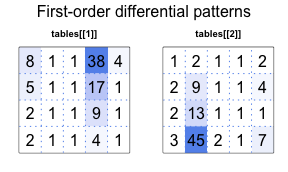cp.chisq.test(tables)
#>
#>  Comparative Chi-Squared Test for Pattern Difference
#>
#> data:  tables
#> X-squared = 199.64, df = 12, p-value < 2.2e-16

sharma.song.test(tables)
#>
#>  Sharma-Song Test for Second-Order Differential Contingency Tables Null
#>  table marginal is observed
#>
#> data:  tables
#> X-squared = 5.6207, df = 12, p-value = 0.934

heterogeneity.test(tables)
#>
#>  Heterogeneity Test for Pattern Difference
#>
#> data:  tables
#> X-squared = 53.413, df = 12, p-value = 3.478e-07

Example 4: Input tables are only second-order differential. At $$\alpha=0.05$$, cp.chisq.test() correctly declares differential patterns; sharma.song.test() correctly declares second-order differential patterns; and heterogenity.test() correctly declares differential patterns.

tables <- list(
matrix(c(
4, 0, 0,
0, 4, 0,
0, 0, 4
), byrow=TRUE, nrow=3),
matrix(c(
0, 4, 4,
4, 0, 4,
4, 4, 0
), byrow=TRUE, nrow=3)
)
par(mfrow=c(1,2), cex=0.5, oma=c(0,0,2,0))
plot_table(tables[], highlight="none", col="salmon", xlab=NA, ylab=NA)
plot_table(tables[], highlight="none", col="salmon", xlab=NA, ylab=NA)
mtext("Second-order differential patterns", outer = TRUE)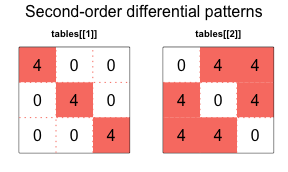cp.chisq.test(tables)
#>
#>  Comparative Chi-Squared Test for Pattern Difference
#>
#> data:  tables
#> X-squared = 36, df = 4, p-value = 2.894e-07

sharma.song.test(tables)
#>
#>  Sharma-Song Test for Second-Order Differential Contingency Tables Null
#>  table marginal is observed
#>
#> data:  tables
#> X-squared = 36, df = 4, p-value = 2.894e-07

heterogeneity.test(tables)
#>
#>  Heterogeneity Test for Pattern Difference
#>
#> data:  tables
#> X-squared = 36, df = 4, p-value = 2.894e-07

Example 5: Input tables are both first- and second-order differential. At $$\alpha=0.05$$, cp.chisq.test() correctly declares differential patterns; sharma.song.test() correctly declares second-order differential patterns; and heterogenity.test() performs incorrectly by not rejecting the tables as having conserved patterns.

tables <- list(
matrix(c(
50,  0, 0,  0,
0,  0, 1,  0,
0, 50, 0,  0,
1,  0, 0,  0,
0,  0, 0, 50
), byrow=T, nrow = 5),
matrix(c(
1,  0,  0, 0,
0,  0, 50, 0,
0,  1,  0, 0,
50,  0,  0, 0,
0,  0,  0, 1
), byrow=T, nrow = 5)
)
par(mfrow=c(1,2), cex=0.5, oma=c(0,0,2,0))
plot_table(tables[], highlight="none", col="orange", xlab=NA, ylab=NA)
plot_table(tables[], highlight="none", col="orange", xlab=NA, ylab=NA)
mtext("Differential patterns", outer = TRUE)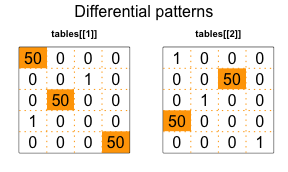cp.chisq.test(tables)
#>
#>  Comparative Chi-Squared Test for Pattern Difference
#>
#> data:  tables
#> X-squared = 919.01, df = 12, p-value < 2.2e-16

sharma.song.test(tables)
#>
#>  Sharma-Song Test for Second-Order Differential Contingency Tables Null
#>  table marginal is observed
#>
#> data:  tables
#> X-squared = 96.239, df = 12, p-value = 3.026e-15

heterogeneity.test(tables)
#>
#>  Heterogeneity Test for Pattern Difference
#>
#> data:  tables
#> X-squared = 0, df = 12, p-value = 1

Conclusions

The examples here demonstrate the use of the package. Most importantly, they also suggest that it may be necessary to consider options different from the default textbook solution to determining heterogeneity across contingency tables.